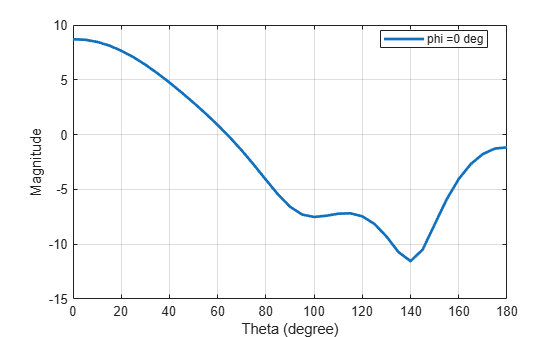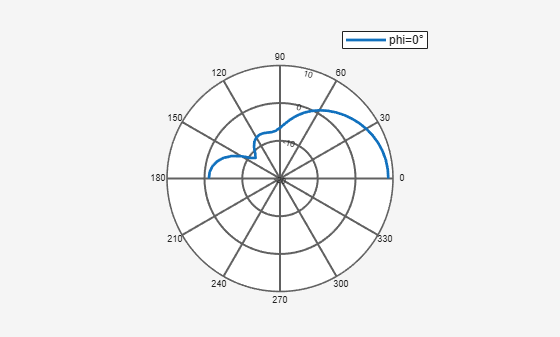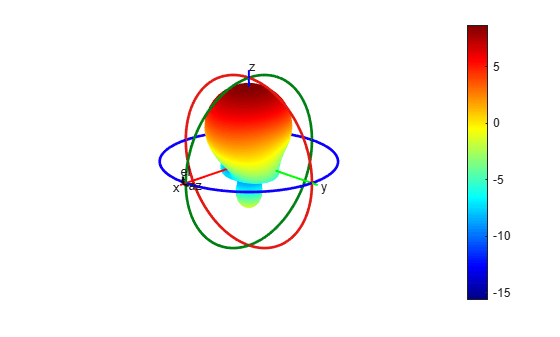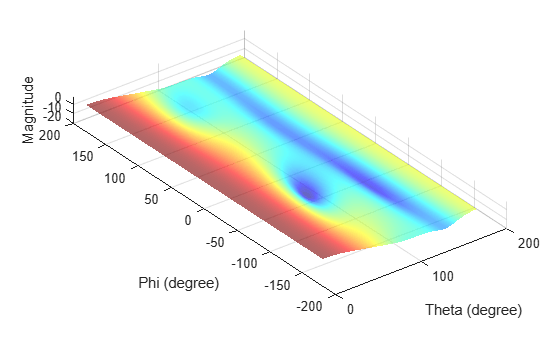# patternCustom

## Syntax

``patternCustom(magE,theta,phi)``
``patternCustom(magE,theta,phi,Name,Value)``
``hplot = patternCustom(___)``

## Description

````patternCustom(magE,theta,phi)` plots the 3-D radiation pattern of an antenna magnitude, `magE` over the specified `phi` and `theta` angle vectors.```

example

````patternCustom(magE,theta,phi,Name,Value)` uses additional options specified by one or more `Name,Value` pair arguments. ```
````hplot = patternCustom(___)` returns handles of the lines or surface in the figure window. This syntax accepts any combination of arguments from the previous syntaxes```

## Examples

### Visualize 3-D Electric Field Pattern of Dipole

Calculate the magnitude, azimuth, and elevation angles of a dipole's electric field at 75 MHz.

```d = dipole; [efield,az,el] = pattern(d, 75e6,'Type','efield');```

Extract the theta and phi angles of the electric field magnitude of the antenna.

```phi = az'; theta = (90-el); MagE = efield';```

Plot the 3-D electric field pattern.

`patternCustom(MagE,theta,phi);`### Visualize 2-D Radiation Patterns of Helix Directivity

Calculate the magnitude, azimuth, and elevation angles of a helix's directivity at 2 GHz.

```h = helix; [D,az,el] = pattern(h,2e9);```

Extract theta and phi angles of the directivity magnitude.

```phi = az'; theta = (90-el); MagE = D';```

Plot 2-D phi slice of the antenna in rectangular coordinates.

```figure; patternCustom(MagE,theta,phi,'CoordinateSystem','rectangular',... 'Slice','phi','SliceValue',0);```Plot 2-D phi slice of the antenna in polar coordinates.

```figure; patternCustom(MagE, theta, phi,'CoordinateSystem','polar',... 'Slice','phi','SliceValue',0);```## Visualize Radiation Pattern From Antenna Data File

Consider a helix antenna data file in .csv format. This file contains the magnitude of the antenna directivity in phi and theta angles. Read the file .

`helixdata = csvread('antennadata_test.csv',1,0);`

Use patternCustom to extract the magnitude of directivity, and the phi, and theta angle values. Plot the 3-D polar radiation pattern.

`patternCustom(helixdata(:,3),helixdata(:,2),helixdata(:,1));`Use the same data to plot the 3-D rectangular radiation pattern.

```figure patternCustom(helixdata(:,3),helixdata(:,2),helixdata(:,1),... 'CoordinateSystem','rectangular');```## Input Arguments

collapse all

Magnitude of plotted quantity, specified as one of the following:

• A N-by-1 real vector . N is the same size as the `phi` and `theta` angle vectors.

• A M-by-R matrix. The matrix should be the same size as `phixtheta`.

where `theta` and `phi` angles are in the spherical coordinate system specified as a vector.

Data quantities plotted include directivity, E-fields, H-fields, or power of an antenna or array object.

Data Types: `double`

Theta angles in spherical coordinates, specified as a vector in degrees.

Data Types: `double`

Phi angles in spherical coordinates, specified as a vector in degrees.

Data Types: `double`

### Name-Value Pair Arguments

Specify optional comma-separated pairs of `Name,Value` arguments. `Name` is the argument name and `Value` is the corresponding value. `Name` must appear inside quotes. You can specify several name and value pair arguments in any order as `Name1,Value1,...,NameN,ValueN`.

Example: `'CoordinateSystem'`,`'rectangular'`

Coordinate system of radiation pattern, specified as the comma-separated pair consisting of `'CoordinateSystem'` and one of these values: `'polar'`, `'rectangular'`.

Example: `'CoordinateSystem'`,`'polar'`

Data Types: `char`

Plane to visualize 2-D data, specified as a comma-separated pair consisting of `'Slice'` and `'theta'` or `'phi'`.

Example: `'Slice'`,`'phi'`

Data Types: `char`

Angle values for slice, specified as a comma-separated pair consisting of `'SliceValue'` and a scalar or a vector.

## Output Arguments

collapse all

Lines or surfaces in figure window, returned as object handle.